# GMAT Math : DSQ: Calculating the diagonal of a cube

## Example Questions

### Example Question #1 : Dsq: Calculating The Diagonal Of A Cube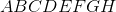is a cube. What is the length of diagonal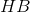?

(1) The area of a face of the cube is.

(2) The length of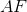is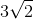.

Statement 2 alone is sufficient.

Statements 1 and 2 taken together are not sufficient.

Each statement alone is sufficient.

Statement 1 alone is sufficient.

Both statements taken together are sufficient.

Each statement alone is sufficient.

Explanation:

To know the diagonal HB of the cube, we need to have information about the length of the edges of the cube. Knowing the area of a face would allow us to find the length of an edge; therefore statement 1 alone is sufficient.

Statment 2 alone is sufficient as well since the length of a diagonal of a square is given by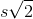, whereis the length of a side of the square and ultimately we can find the length of an edge of the square.

Therefore each statement alone is sufficient.

### Example Question #2 : Dsq: Calculating The Diagonal Of A Cube

Calculate the diagonal of a cube.

1. The surface area of the cube is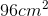.
2. The volume of the cube is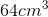.

Statements 1 and 2 are not sufficient, and additional data is needed to answer the question.

Each statement alone is sufficient to answer the question.

Statement 2 alone is sufficient, but statement 1 alone is not sufficient to answer the question.

Statement 1 alone is sufficient, but statement 2 alone is not sufficient to answer the question.

Both statements taken together are sufficient to answer the question, but neither statement alone is sufficient.

Each statement alone is sufficient to answer the question.

Explanation:

Statement 1: We can use the surface area to find the length of the cube's edge and then determine the length of the diagonal.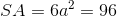whererepresents the length of the cube's edge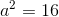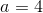Now that we know the length of the edge, we can find the length of the diagonal: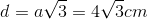.

Statement 2: Once again, we can use the provided information to find the length of the cube's edge.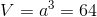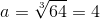Which means the length of the diagonal is.

Thus, each statement alone is sufficient to answer the question.

### Example Question #3 : Dsq: Calculating The Diagonal Of A Cube

Give the length of the diagonal of the cube.

1. The surface area of the cube is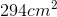.
2. The volume of the cube is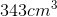.

Each statement alone is sufficient to answer the question.

Both statements taken together are sufficient to answer the question, but neither statement alone is sufficient.

Statements 1 and 2 are not sufficient, and additional data is needed to answer the question.

Statement 2 alone is sufficient, but statement 1 alone is not sufficient to answer the question.

Statement 1 alone is sufficient, but statement 2 alone is not sufficient to answer the question.

Each statement alone is sufficient to answer the question.

Explanation:

Statement 1: To find the length of the diagonal of the cube we need to find the length of the cube's edge. We can use the given surface area value to do so: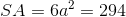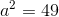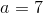Now that we know the length of the cube's edge, we can calculate the diagonal: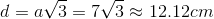Statement 2: We're given the volume of the cube which we can also use to solve for the length of the cube's edge.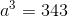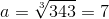Knowing the length of the cube's edge allows us to calculate the diagonal:Each statement alone is sufficient to answer the question.

### Example Question #4 : Dsq: Calculating The Diagonal Of A Cube

Find the diagonal of the cube.

1. The surface area of the cube is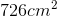.
2. The length of an edge of the cube measures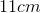.

Each statement alone is sufficient to answer the question.

Both statements taken together are sufficient to answer the question, but neither statement alone is sufficient.

Statement 1 alone is sufficient, but statement 2 alone is not sufficient to answer the question.

Statements 1 and 2 are not sufficient, and additional data is needed to answer the question.

Statement 2 alone is sufficient, but statement 1 alone is not sufficient to answer the question.

Each statement alone is sufficient to answer the question.

Explanation:

Statement 1: We need the length of an edge in order to find the diagonal of the cube. Luckily, we can find the length using the information provided: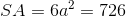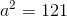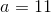Now that we know the length of an edge is 11 cm we can find the length of the diagonal.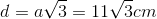Statement 2: We're given the information we need to find the diagonal, all we need to do is plug it into the equation### Example Question #5 : Dsq: Calculating The Diagonal Of A Cube

What is the length of the diagonal of the cube A if the diagonal of cube B is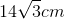?

1. The lengths of the edges of cube A to cube B is a ratio of 1:2.
2. The surface area of cube A is.

Statements 1 and 2 are not sufficient, and additional data is needed to answer the question.

Statement 1 alone is sufficient, but statement 2 alone is not sufficient to answer the question.

Each statement alone is sufficient to answer the question.

Both statements taken together are sufficient to answer the question, but neither statement alone is sufficient.

Statement 2 alone is sufficient, but statement 1 alone is not sufficient to answer the question.

Each statement alone is sufficient to answer the question.

Explanation:

Statement 1: The information provided in the question is only useful if we're given a relationship between cube A and cube B. Since this statement does provide us with the ratio of 1:2, we can answer the question.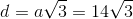whererepresents the length of the cube's edge

we can easily see the length measures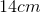Remember the ratio of cube A to cube B is 1:2.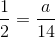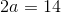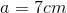Now that we know the length, we can find the diagonal of the cube: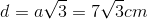Statement 2: We're given information about cube A so we don't need to worry about cube B. Using this information we can solve for the edge length of cube A and then calculate the diagonal.Knowing the length of the edge allows us to find the diagonal of the cubeTired of practice problems?

Try live online GMAT prep today.